# Oops...

This website is made for modern browsers. You are seeing this, because your browser is missing a feature or two. Please install the latest update or switch to a modern browser. See you soon.

# Tapered girder grid

In this tutorial, we have a look at different functions that involve numeric domains. To illustrate this topic, we will create a tapered girder grid.

## Grasshopper

#### 1

##### Create basic grid

First thing we do, is to define the number of girders that we want to have in each direction. This number is written in a PanelPanel
Params  >  Input  >  Panel
A panel for custom notes and text values
(here 10). The component Square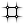Square (SqGrid)
Vector  >  Grid  >  Square
2D grid with square cells
Inputs
Plane (P)Base plane for grid
Size (S)Size of grid cells
Extent X (Ex)Number of grid cells in base plane x direction
Extent Y (Ey)Number of grid cells in base plane y direction
Outputs
Cells (C)Grid cell outlines
Points (P)Points at grid corners
is used to create the basic grid: At its inputs Ex and Ey we set the number of grid cells, not the number of grid lines. Therefore, we have to subtract 1 from our number of girders. For this, we use a Subtraction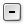Subtraction (A-B)
Maths  >  Operators  >  Subtraction
Mathematical subtraction
Inputs
A (A)First operand for subtraction
B (B)Second operand for subtraction
Outputs
Result (R)Result of subtraction
component. The size S of the grid cells can be set to an arbitrary number.

#### 2

##### Set curves for the sag

The tapering of the girders will not be same for each individual girder, but instead the network of girders should correspond to a sagging surface. The sag or the form of the surface will follow a sine curve in every cross-section. This will be controlled by offsetting every node of the grid and thus defining the bottom chord of the girders.Finding the bottom chord by moving the top nodes down.

Each girder is segmented by the girders running in the perpendicular direction and we need the vertical coordinate according to the sine curve for each intersection. To do this, we need an array that has the same length as the number of girders in one direction. This is achieved with a SeriesSeries (Series)
Sets  >  Sequence  >  Series
Create a series of numbers.
Inputs
Start (S)First number in the series
Step (N)Step size for each successive number
Count (C)Number of values in the series
Outputs
Series (S)Series of numbers
component at which we input the number of girders at input C.

A sine curve can be drawn with the Graph Mapper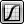Graph Mapper (Graph)
Params  >  Input  >  Graph Mapper
Represents a numeric mapping function
component. After placing the component on the canvas, we right-click it and select Graph types > Sine in the context menu. The component will now display a quarter of a sine curve and we need to adjust it to show half of a sine curve (0 To Pi). This is done by moving the top handle towards the middle until the curve is centered and symmetrical (yes, this is impossible but zooming in will help).Graph Mapper

The default range of the Graph Mapper’s coordinates is from 0 to 1 on each axis and it’s advised to leave it like that and rather apply a factor to the output to scale to the desired magnitude. And of course, if we alter the internal coordinates, we also have to provide input data in this range.

The output of our Series is not within the needed range and we use Remap Numbers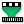Remap Numbers (ReMap)
Maths  >  Domain  >  Remap Numbers
Remap numbers into a new numeric domain
Inputs
Value (V)Value to remap
Source (S)Source domain
Target (T)Target domain
Outputs
Mapped (R)Remapped number
Clipped (C)Remapped and clipped number
to convert it: Input S takes the source domain, which we get from using a Bounds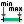Bounds (Bnd)
Maths  >  Domain  >  Bounds
Create a numeric domain which encompasses a list of numbers.
Inputs
Numbers (N)Numbers to include in Bounds
Outputs
Domain (I)Numeric Domain between the lowest and highest numbers in {N}
component. Input T takes the target domain, which by default is already 0 To 1. The values to be remapped are set at input V and to which we connect our Series. The remapped series are now the input (x-values) for the Graph Mapper.

#### 3

##### Get sag values for the surface

The output (y-values) of the Graph MapperGraph Mapper (Graph)
Params  >  Input  >  Graph Mapper
Represents a numeric mapping function
is within the range of the domain 0 To 1 and we need to remap the values to the desired magnitude before they define the bottom chord of our girders. Thus, we us Remap NumbersRemap Numbers (ReMap)
Maths  >  Domain  >  Remap Numbers
Remap numbers into a new numeric domain
Inputs
Value (V)Value to remap
Source (S)Source domain
Target (T)Target domain
Outputs
Mapped (R)Remapped number
Clipped (C)Remapped and clipped number
again. This time, the default source domain of 0 To 1 is correct and we need a target domain.

To define the target domain we use Construct Domain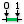Construct Domain (Dom)
Maths  >  Domain  >  Construct Domain
Create a numeric domain from two numeric extremes.
Inputs
Domain start (A)Start value of numeric domain
Domain end (B)End value of numeric domain
Outputs
Domain (I)Numeric domain between {A} and {B}
and connect a Number Slider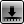Number Slider
Params  >  Input  >  Number Slider
Numeric slider for single values
to each input. Because these values will define the bottom chord in relation to the grid points, we use negative values to later get a vector pointing downwards. A shortcut to create such a slider is double-clicking on the canvas and typing -2.0..-0.5 into the canvas search.

#### 4

##### Get tapering values for all points

So far, we have obtained values for the sag of the surface, or more precisely, for the cross-section in the middle. When describing this surface with a grid of tapered girders, the girders towards the edges must be less tapered than the ones in the middle. Therefore, each girder needs to have the same height at the edge of the surface, but a different height in its respective middle.Each girder is created with a different amplitude of the sine curve.

To implement this, we use another Construct DomainConstruct Domain (Dom)
Maths  >  Domain  >  Construct Domain
Create a numeric domain from two numeric extremes.
Inputs
Domain start (A)Start value of numeric domain
Domain end (B)End value of numeric domain
Outputs
Domain (I)Numeric domain between {A} and {B}
component and connect the smaller height to input A (here -0.5). We also connect the output of the remapped values from step 3 to input B. We now have domains in which the lower bound is the height for the end points of a girder and the upper bound is the height in the middle.

These domains will now become the target domain T of another Remap NumbersRemap Numbers (ReMap)
Maths  >  Domain  >  Remap Numbers
Remap numbers into a new numeric domain
Inputs
Value (V)Value to remap
Source (S)Source domain
Target (T)Target domain
Outputs
Mapped (R)Remapped number
Clipped (C)Remapped and clipped number
component and we connect the output of the Graph Mapper to input V. The values from the sine curve will now be remapped within the new domains, but because the upper bounds change, we get smoother (less curved) curves at the edge area. Also, we need to Graft input T to handle the two dimensions of the girder gird; to get tapering in both directions.

#### 5

##### Move points accordingly

After generating the heights, we will connect them to a Unit Z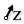Unit Z (Z)
Vector  >  Vector  >  Unit Z
Unit vector parallel to the world {z} axis.
Inputs
Factor (F)Unit multiplication
Outputs
Unit vector (V)World {z} vector
component which turns them into vectors. Then, we use these vectors to Move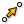Move (Move)
Transform  >  Euclidean  >  Move
Translate (move) an object along a vector.
Inputs
Geometry (G)Base geometry
Motion (T)Translation vector
Outputs
Geometry (G)Translated geometry
Transform (X)Transformation data
the points of the Square grid that we created in step 1.

#### 6

##### Arrange points in rows and columns

The grid points are organized in rows, but we also need the points in the column direction. To get them, we connect a Flip Matrix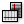Flip Matrix (Flip)
Sets  >  Tree  >  Flip Matrix
Flip a matrix-like data tree by swapping rows and columns.
Inputs
Data (D)Data matrix to flip
Outputs
Data (D)Flipped data matrix
component to the points of the top grid and to the ones of the bottom grid. Next, an Entwine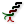Entwine (Entwine)
Sets  >  Tree  >  Entwine
Flatten and combine a collection of data streams
Inputs
Branch {0;0} ({0;0})Data to entwine
Branch {0;1} ({0;1})Data to entwine
Branch {0;2} ({0;2})Data to entwine
Outputs
Result (R)Entwined result
component will sort each direction into a separate branch of a data tree
This page is not finished yet.
. But for this to happen, we need to right-click the component and deselect Flatten Inputs in the context menu.

#### 7

##### Generate outlines and surfaces

Now, that we have all needed points and did the sorting, we connect a PolyLine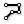PolyLine (PLine)
Curve  >  Spline  >  PolyLine
Create a polyline connecting a number of points.
Inputs
Vertices (V)Polyline vertex points
Closed (C)Close polyline
Outputs
Polyline (Pl)Resulting polyline
component to the points of the top grid and an Interpolate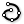Interpolate (IntCrv)
Curve  >  Spline  >  Interpolate
Create an interpolated curve through a set of points.
Inputs
Vertices (V)Interpolation points
Degree (D)Curve degree
Periodic (P)Periodic curve
KnotStyle (K)Knot spacing (0=uniform, 1=chord, 2=sqrtchord)
Outputs
Curve (C)Resulting nurbs curve
Length (L)Curve length
Domain (D)Curve domain
component to the points of the bottom grid. This will generate curves for the top and bottom edges of the girders. The component Ruled Surface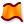Ruled Surface (RuleSrf)
Surface  >  Freeform  >  Ruled Surface
Create a surface between two curves.
Inputs
Curve A (A)First curve
Curve B (B)Second curve
Outputs
Surface (S)Ruled surface between A and B
then takes all curves as input and will create a surface between each pair of curves. This works as desired thanks to the data tree structure that we provided.

#### 8

##### Create tapered volumes as girders

The last step of this tutorial is to turn the surfaces into volumes. This is done with an Extrude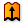Extrude (Extr)
Surface  >  Freeform  >  Extrude
Extrude curves and surfaces along a vector.
Inputs
Base (B)Profile curve or surface
Direction (D)Extrusion direction
Outputs
Extrusion (E)Extrusion result
component, at which we have to provide the direction for each extrusion at input D. Each surface will be extruded in the direction of the surface’s normal. We find the normals at given uv-coordinates with an Evaluate Surface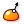Evaluate Surface (EvalSrf)
Surface  >  Analysis  >  Evaluate Surface
Evaluate local surface properties at a {uv} coordinate.
Inputs
Surface (S)Base surface
Point (uv){uv} coordinate to evaluate
Outputs
Point (P)Point at {uv}
Normal (N)Normal at {uv}
U direction (U)U direction at {uv}
V direction (V)V direction at {uv}
Frame (F)Frame at {uv}
component. Here we use 0,0 as uv-values, but any value pair will work, because we have planar surfaces.

The normals are outputted at N and we append an Amplitude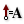Amplitude (Amp)
Vector  >  Vector  >  Amplitude
Set the amplitude (length) of a vector.
Inputs
Vector (V)Base vector
Amplitude (A)Amplitude (length) value
Outputs
Vector (V)Resulting vector
component. The thickness of the extrusion can be regulated with a Number SliderNumber Slider
Params  >  Input  >  Number Slider
Numeric slider for single values
at input A. The output V is connected to input D of the Extrude component and now we have created three-dimensional, tapered girders.

## Get the results

### Version Info

• Rhino 6.30
• Grasshopper 1.0.0007

## Test your skills

The solution above works fine, but it doesn’t give us the smoothest results, because the beams intersect. To get nicer results and also to let the bottoms align with the sagging surface at the intersections, we can create the sagging surface first and then cut the girders out. Thus, instead of the beams, we need a surface for the top and a second one for the button. Also, we need some boxes to trim holes into the surfaces. Enough said, now it’s your turn to try this approach yourself.

Hint 1

### Create two surfaces

From the solution above, we can keep everything until after step 5. But, instead of creating curves for the girders, we create surfaces from the grid of points. The component Surface From Points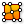Surface From Points (SrfGrid)
Surface  >  Freeform  >  Surface From Points
Create a nurbs surface from a grid of points.
Inputs
Points (P)Grid of points
U Count (U)Number of points in {u} direction
Interpolate (I)Interpolate samples
Outputs
Surface (S)Resulting surface
is what we need, but we have to eliminate the data tree structure
This page is not finished yet.
by flattening input P. At input U, we provide the number of points in one direction. This is the number of beams which we originally defined in step 1. Next, we need boxes to cut holes into the surfaces.

Hint 2

### Create cutting boxes

We create a box in each cell that will serve as trimming objects for the surfaces. We start with defining the boxes right after step 1, where we got the grid cell outlines from output C of the Square component. The center of each cell is then generated with an Area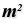Area (Area)
Surface  >  Analysis  >  Area
Solve area properties for breps, meshes and planar closed curves.
Inputs
Geometry (G)Brep, mesh or planar closed curve for area computation
Outputs
Area (A)Area of geometry
Centroid (C)Area centroid of geometry
component and we connect its output C with a Center Box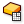Center Box (Box)
Surface  >  Primitive  >  Center Box
Create a box centered on a plane.
Inputs
Base (B)Base plane
X (X)Size of box in {x} direction.
Y (Y)Size of box in {y} direction.
Z (Z)Size of box in {z} direction.
Outputs
Box (B)Resulting box
component. These boxes do not only need to have a center point (converted to a base plane) at input B, but also dimensions in each direction.

The height of the boxes should not be smaller than the tallest girder. This value was defined by the Number Slider of the Domain end in step 2 and we can grab it from there. The expansion of the boxes in x- and y-direction should be half of each cell size minus half of the girder width. To calculate this, we use an Expression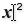Expression (Expression)
Maths  >  Script  >  Expression
Evaluate an expression
Inputs
Variable x (x)Expression variable
Variable y (y)Expression variable
Outputs
Result (R)Result of expression
component and set the expression to (y-x)/2. At input x we connect a Number SliderNumber Slider
Params  >  Input  >  Number Slider
Numeric slider for single values
for a variable girder width and at input y we connect the size of the grid cells that we defined in step 1. Now, you should be able to use these boxes to cut holes into the previously created surfaces.

Hint 3

### Trim surfaces

To cut holes into the surfaces, we use the component Trim Solid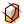Trim Solid (Trim)
Intersect  >  Shape  >  Trim Solid
Cut holes into a shape with a set of solid cutters.
Inputs
Shape (S)Shape to trim
Cutters (T)Trimming shapes
Outputs
Result (R)Shape with holes
. Here, we connect the surface for the trim at input S and the trimming shapes, our boxes, at input T. The shapes must be provided as a list, and so we have to flatten the second input which removes the data tree structure. Now, we have the top and bottom surface of our girder grid. But, side surfaces are still missing.

Hint 4

### Create missing surfaces

To close the missing sides, we create surfaces between the edges of the top and bottom surface. These edges can be extracted with the Brep Edges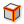Brep Edges (Edges)
Surface  >  Analysis  >  Brep Edges
Extract the edge curves of a brep.
Inputs
Brep (B)Base Brep
Outputs
Naked (En)Naked edge curves
Interior (Ei)Interior edge curves
Non-Manifold (Em)Non-Manifold edge curves
component and then connected with a Ruled SurfaceRuled Surface (RuleSrf)
Surface  >  Freeform  >  Ruled Surface
Create a surface between two curves.
Inputs
Curve A (A)First curve
Curve B (B)Second curve
Outputs
Surface (S)Ruled surface between A and B
component. To connect the correct edges, we have to modify the data structure by grafting both inputs of the Ruled Surface component. Finally, we can combine all surfaces of the girder grid with a Brep Join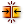Brep Join (Join)
Surface  >  Util  >  Brep Join
Join a number of Breps together
Inputs
Breps (B)Breps to join
Outputs
Breps (B)Joined Breps
Closed (C)Closed flag for each resulting Brep
component, on which we flatten the input. This way, we receive a smooth grid object.

Get the results

## Get the results

### Version Info

• Rhino 6.30
• Grasshopper 1.0.0007

### Download Files

This page is open source. Edit it on GitHub or see how you can contribute.